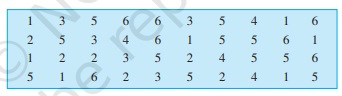# Catherine threw a dice 40 times and noted the number appearing each time as shown below:Make a table and enter the data using tally marks. Find the number that appeared.(a) The minimum number of times(b) The maximum number of times(c) Find those numbers that appear an equal number of times."

To do:

We have to make a table and enter the data using tally marks and find the number that appeared.

(a) The minimum number of times
(b) The maximum number of times
(c)  An equal number of times.

Solution:

(a) The required tally marks table is as follows

 Numbers Tally Marks Number of times 1 |||| || 7 2 |||| | 6 3 |||| 5 4 |||| 4 5 |||| |||| | 11 6 |||| || 7

(a) The number that occurred the minimum number of times is 4.

(b) The number that occurred the maximum number of times is 5.

(c) 1 and 6 are the numbers that appear an equal number of times.